Study P4 Mathematics Maths - Area and Perimeter - Geniebook# Area And Perimeter 1

1. Finding the maximum number of squares that can be fitted/cut from a rectangle
2. Finding unknown dimensions given area of a rectangle/square
3. Finding unknown dimensions given perimeter of a rectangle/square

Let’s recap P3 Area and Perimeter first!

## Perimeter

The perimeter of a shape is the total distance around the shape.

### Perimeter Of A Square

 \begin{align} &= \text{Length + Length + Length + Length} \\[2ex] &= 4 \times \text{Length} \end{align}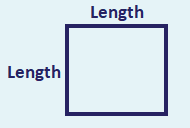### Perimeter Of A Rectangle

 $$= \text{Length + Breadth + Length + Breadth}$$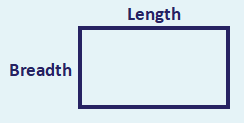## Area

The area is the space occupied by the figure.

### Area Of A Square

 \begin{align} = \text{Length} \times \text{Length} \end{align}### Area Of A Rectangle

 \begin{align} = \text{Length} \times \text{Breadth} \end{align}Question 1:

Find the area and perimeter of the square below.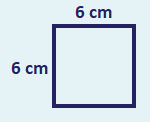Solution:

Area of square

\begin{align} &= 6 \;cm \times 6 \;cm \\[2ex] &= 36 \;cm^2 \end{align}

Perimeter of square

\begin{align} &= 4 \times 6 \;cm \\[2ex] &= 24 \;cm \end{align}

Area: $$36 \;cm^2$$

Perimeter: $$24 \;cm$$

Question 2:

Find the area and perimeter of the rectangle below.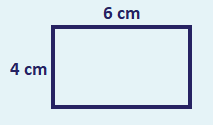Solution:

Area of rectangle

\begin{align} &= 6 \;cm \times 4 \;cm \\[2ex] &= 24 \;cm^2 \end{align}

Perimeter of rectangle

\begin{align} &= 6 \;cm + 4 \;cm + 6 \;cm + 4 \;cm \\[2ex] &= 20 \;cm \end{align}

Area: $$24 \;cm^2$$

Perimeter: $$20 \;cm$$

## 1. Finding the maximum number of squares that can be     fitted / cut from a rectangle

To find the maximum number of squares that can be fitted/cut from an area, we will first find out the number of squares that are able to fit along the length and the breadth of that area.

Question 1:

What is the maximum number of $$1 \;cm$$ squares that can be cut from the rectangle?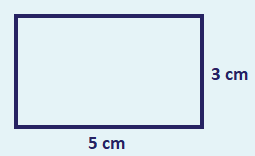Solution: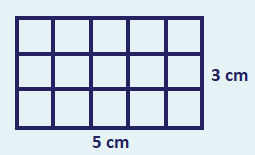Number of $$1 \;cm$$ squares along the length of the rectangle

\begin{align} &= 5 \;cm ÷ 1 \;cm \\[2ex] &= 5 \end{align} ​

Number of $$1 \;cm$$ squares along the breadth of the rectangle

\begin{align} &= 3 \;cm ÷ 1 \;cm \\[2ex] &= 3 \end{align} ​

Maximum number of squares that can be cut from the rectangle

\begin{align} &= 5 \times 3 \\[2ex] &= 15 \end{align}

$$15$$ squares

Question 2:

What is the maximum number of $$2 \;cm$$ squares that can be cut from the rectangle?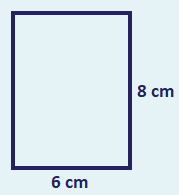Solution: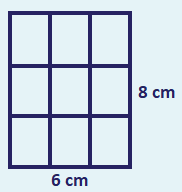Number of $$2 \;cm$$ squares along the length of the rectangle

\begin{align} &= 8 \;cm \div 2 \;cm \\[2ex] &= 4 \end{align}

Number of $$2 \;cm$$ squares along the breadth of the rectangle

\begin{align} &= 6 \;cm \div 2 \;cm \\[2ex] &= 3 \end{align}

Maximum number of squares that can be cut from the rectangle

\begin{align} &= 4 \times 3 \\[2ex] &= 12 \end{align}

$$12$$ squares

Question 3:

What is the greatest number of 4-cm squares that can be cut from the rectangle?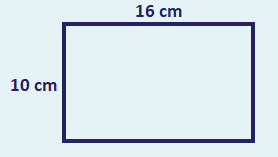Solution: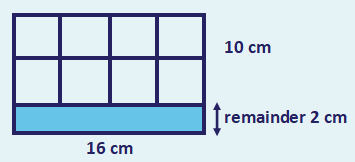Number of $$4 \;cm$$ squares along the length of the rectangle

\begin{align} &= 16 \;cm \div 4\;cm \\[2ex] &= 4 \end{align}

Number of $$4 \;cm$$ squares along the breadth of the rectangle

\begin{align} &= 10 \;cm \div 4\;cm \\[2ex] &= 2\;R \;2\;cm \end{align}

We ignore the part which is represented by the remainder of $$2\;cm$$ as no squares can be cut from it.

Greatest number of squares that can be cut from the rectangle

\begin{align} &= 4 \times 2 \\[2ex] &= 8 \end{align}

$$8$$ squares

## 2. Finding unknown dimensions given area of a rectangle / square

$$\text{Area Of A Rectangle} = \text{Length} \times \text{Breadth}$$

Therefore,

\begin{align} \text{Length Of A Rectangle} &= \text{Area} \div \text{Breadth} \\[2ex] \text{Breadth Of A Rectangle} &= \text{Area} \div \text{Length} \end{align} ​

Question 1:

The area of a rectangle is $$126 \;cm^2$$. If its breadth is $$7 \;cm$$, what is the length of the rectangle?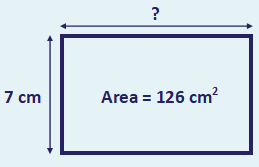Solution:

Length of the rectangle

\begin{align} &= 126 \;cm^2 ÷ 7 \;cm \\[2ex] &= 18 \;cm \end{align}

$$18 \;cm$$

Question 2:

The area of a rectangle is 72 cm2. Given that the length of the rectangle is 9 cm, find the breadth of the rectangle.Solution:

\begin{align}​​ &= 72 \;cm^2 \div 9 \;cm\\[2ex] &= 8 \;cm \end{align}

$$8 \;cm$$

Question 3:

The area of a square is $$64 \;cm^2$$. Find the length of one side of the square.Solution:

Since $$8 \;cm \times 8 \;cm = 64 \;cm^2$$,

Length of one side of each square $$= 8 \;cm$$

$$8 \;cm$$

Question 4:

The figure below is made up of $$3$$ identical squares. Given that the total area of the figure is $$75 \;cm^2$$, find the length of one side of each square.Solution:

Area of 1 square

\begin{align}​​ &= 75 \;cm^2 \div 3\\[2ex] &= 25 \;cm^2 \end{align}

Since $$5 \;cm \times 5 \;cm = 25 \;cm^2$$,

Length of one side of each square $$= 5 \;cm$$

$$5 \;cm$$

## 3. Finding unknown dimensions given perimeter of a rectangle/square

\begin{align} \text{Perimeter Of Rectangle} &= \text{Length + Length + Breadth + Breadth}\\[2ex] \text{Length Of Rectangle} &= \text{(Perimeter - Breadth - Breadth) ÷ 2}\\[2ex] \text{Breadth Of Rectangle} &= \text{(Perimeter - Length - Length) ÷ 2} \end{align}

Question 1:

The perimeter of a rectangle is $$36 \;cm$$. Given that its breadth is $$5 \;cm$$, find its length.Solution:

\begin{align}​​ \text{Perimeter of a rectangle} &= \text{Length + Breadth + Length + Breadth} \\[3ex] \text{Total length of 2 lengths} &= 36 \;cm - 5 \;cm - 5 \;cm \\[2ex] &= 26 \;cm\\[3ex] \text{Length of rectangle} &= 26 \;cm ÷ 2 \\[2ex] &= 13 \;cm \end{align}

$$13 \;cm$$

Question 2:

The perimeter of a rectangle is $$72 \;cm$$. Given that its length is $$24 \;cm$$, find its breadth.Solution:

\begin{align}​​ \text{Perimeter of a rectangle} &= \text{Length + Breadth + Length + Breadth} \\[3ex] \text{Total length of 2 breadths} &= 72 \;cm - 24 \;cm - 24 \;cm \\[2ex] &= 24 \;cm\\[3ex] \text{ Breadth of rectangle} &= 24 \;cm ÷ 2 \\[2ex] &= 12 \;cm \end{align}

$$12 \;cm$$

​\begin{align} \text{Perimeter of a square} &= 4 \times \text{Length} \\[2ex] \text{Length of one side of a square} &= \text{Perimeter} \div 4 \end{align}

Question 3:

The perimeter of a square is $$60 \;cm$$. Find the length of one side of the square.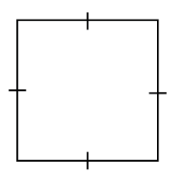Solution:

Perimeter of a square $$= 4 \;×$$ Length
Length of one side of the square\begin{align}​​\\[2ex] &= 60 \;cm \div 4\\[2ex] &= 15 \;cm \end{align}

$$15 \;cm$$

Question 4:

The area of a rectangular garden is $$168 \;m^2$$. Its breadth is 8 m.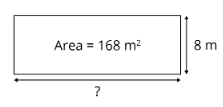1. Find the length of the garden.
2. Vincent jogged round the entire rectangular garden twice. Find the distance he jogged.

Solution:

1. Length of the rectangle\begin{align}​​\\[2ex] &= 168 \;m^2 \div 8 \;m \\[2ex] &= 21 \;m \end{align}
2. Perimeter of garden\begin{align}​​\\[2ex] &= 21 \;m + 8 \;m + 21 \;m + 8 \;m \\[2ex] &= 58 \;m \end{align}
Distance he jogged \begin{align}​​\\[2ex] &= 58 \;m \times 2\\[2ex] &= 116 \;m \end{align}

1. $$21 \;m$$

1. $$116 \;m$$

Question 5:

Elaine jogged $$36 \;m$$ round a square sand pit.1. Find the length of one side of the sand pit.
2. Find the area of the square sand pit.

Solution:

1. Length of one side of the sand pit\begin{align}​​\\[2ex] &= 36 \;m \div 4\\[2ex] &= 9 \;m \end{align}
2. Area of the sand pit\begin{align}​​\\[2ex] &= 9 \;m \times 9 \;m\\[2ex] &= 81 \;m^2 \end{align}

1. $$9 \;m$$

1. $$81 \;m^2$$

Continue Learning
Multiplication Whole Numbers
Multiplication And Division Decimals
Model Drawing Strategy Division
Fractions Factors And Multiples
Area And Perimeter 1 Line Graphs
TimePrimaryPrimary 1Primary 2Primary 3Primary 4EnglishMaths
Multiplication
Whole Numbers
Line Graphs
Time
Multiplication And Division
Decimals
Model Drawing Strategy
Division
Fractions
Factors And Multiples
Area And Perimeter 1SciencePrimary 5Primary 6SecondarySecondary 1Secondary 2Secondary 3Secondary 4